Subsections

# Basic quantum operations

To manipulate quantum registers and to implement quantum algorithms, libquantum provides a set of function for quantum register manipulation by quantum gates. Each gate is called via a common interface:

 quantum_NAME(QUBIT[S], [PARAMETER], ®ISTER);

NAME is the name of the gate, QUBIT[S] are one ore more integers describing the Qubits that are relevant for the operation. All qubits are given in a notation starting with 0 as the least significant bit. Some functions require an additional PARAMETER, e.g. an angle of a rotation. &REGISTER contains the address of the quantum register.

## quantum_cnot

 extern void quantum_cnot(int control, int target, quantum_reg *reg);

This function performs a controlled not operation. The target bit gets inverted if the control bit is enabled. The operation can be written as the unitary operation matrix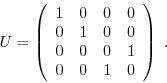## quantum_toffoli

 extern void quantum_toffoli(int control1, int control2, int target, quantum_reg *reg);

This operation is a controlled-not with two controlling qubits. The unitary operation matrix is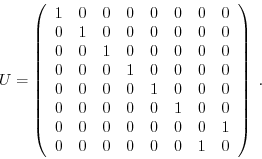## quantum_unbounded_toffoli

 extern void quantum_unbounded_toffoli(int controlling, quantum_reg *reg, ...);

An unbounded Toffoli gate is a controlled-not operation with an arbritrary number of controlling qubits. This gate is not considered elementary and is not available on all physical realizations of a quantum computer. Be sure that controlling contains the correct number of controlling qubits. The last argument contains the target qubit.

## quantum_sigma_x, quantum_sigma_y, quantum_sigma_z

 extern void quantum_sigma_x(int target, quantum_reg *reg); extern void quantum_sigma_y(int target, quantum_reg *reg); extern void quantum_sigma_z(int target, quantum_reg *reg);

These functions perform the Pauli spin operations, defined as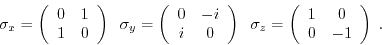Note that the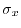operation is the same as a not operation.

## quantum_r_x, quantum_r_y, quantum_r_z

 extern void quantum_r_x(int target, float gamma, quantum_reg *reg); extern void quantum_r_y(int target, float gamma, quantum_reg *reg); extern void quantum_r_z(int target, float gamma, quantum_reg *reg);

These functions perform a rotation of a qubit about an axis of the Bloch sphere. The angle of the rotation is given by gamma. The rotations can be described by their unitary operation matrices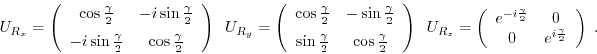## quantum_phase_scale

 extern void quantum_phase_scale(int target, float gamma, quantum_reg *reg);

This function adds a global phase on a qubit, as shown in its unitary operation matrix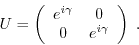## quantum_phase_kick

 extern void quantum_phase_kick(int target, float gamma, quantum_reg *reg);

This function performs a phase kick or phase shift. It is described by the unitary operation matrix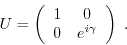extern void quantum_hadamard(int target, quantum_reg *reg);

This function provides the widely used Hadamard gate. The Hadamard gate can establish or destroy the superposition of a qubit. The unitary operation matrix is defined as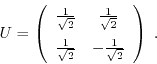## quantum_walsh

 extern void quantum_walsh(int width, quantum_reg *reg);

The Walsh-Hadamard transform consists of Hadamard gates applied on the first width qubits of the quantum register.

## quantum_cond_phase

 extern void quantum_cond_phase(int control, int target, quantum_reg *reg);

This function performs a conditional phase shift. It is a controlled phase kick about the angle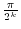with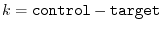. The unitary operation matrix is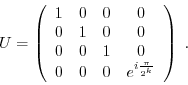## quantum_cond_phase_kick

 extern void quantum_cond_phase_kick(int control, int target, float gamma, quantum_reg *reg);

This function does a phase kick on the target bit about the angle gamma, controlled by control. The unitary operation matrix is defined as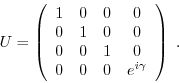## quantum_gate1

 extern void quantum_gate1(int target, quantum_matrix m, quantum_reg *reg);

This function provides an interface for arbitrary 1-bit operations. The operation matrix is given in the following structure:

 quantum_matrix {    int rows;    int cols;    COMPLEX_FLOAT *t; };

The elements of a matrix m are arranged as follows: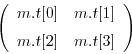New matrices can be created using quantum_new_matrix:

 extern quantum_matrix quantum_new_matrix(int cols, int rows);

To delete a matrix and free its allocated memory, use quantum_delete_matrix:

 extern void quantum_delete_matrix(quantum_matrix *m);

## quantum_gate2

 extern void quantum_gate2(int target1, int target2, quantum_matrix m, quantum_reg *reg);

Provides arbitrary two-bit gates. The parameters are identical to those to quantum_gate1, except that the matrix acts on two qubits, target1 and target2.